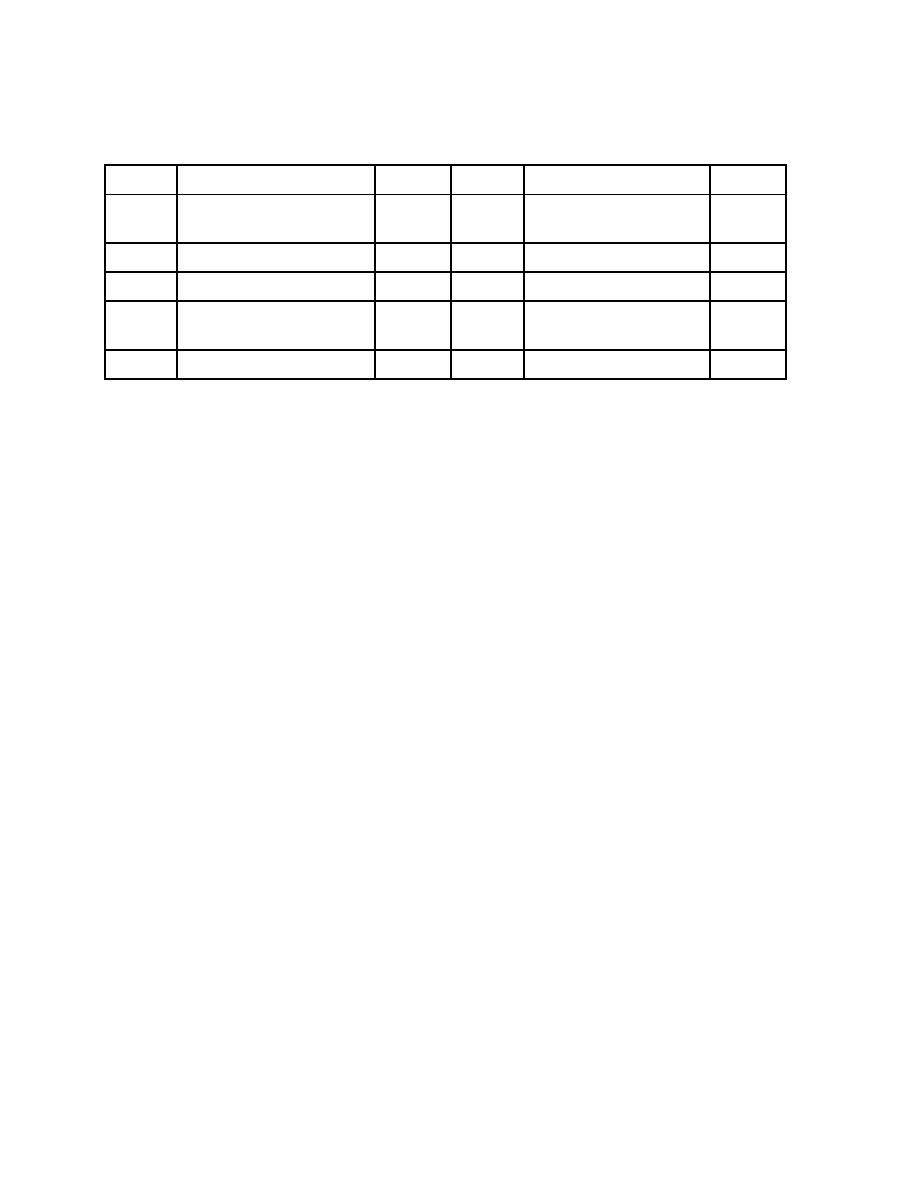Click here to make tpub.com your Home Page Page Title: Table B-3. Values of Parameters used to Calculate Pressure Back | Up | NextWeb www.tpub.comHome

Information Categories
Aerographer
Automotive
Aviation
Construction
Diving
Draftsman
Engineering
Electronics
Food and Cooking
Logistics
Math
Medical
Music
Nuclear Fundamentals
Photography
ReligionDOE-STD-3013-2004
Table B-3
Values of Parameters used to Calculate Pressure
Symbol
Quantity
Value
Symbol
Quantity
Value
P
Container Pressure
psia
V1
Gas Volume of
1.704 l
Container*
P0
Fill Gas Pressure at Filling 14.7 psia
m
Mass of Oxide
5.0 kg
ρ
T1
Evaluation Temperature
477.4 K
Density of Oxide
10 kg/l
T0
Fill Gas Temperature at
303 K
Q0
Container Energy
12.4 w
Filling
Generation
τ
L
Water Content
0.5 wt%
Storage Time
50 yr.
* The gas volume is determined by subtracting the material volume (mass of 5.0 kg divided by density of
10 kg/l to yield 0.5 l) from the 2.204 l free volume of the BNFL container system.
P = P0(T1/T0).+ 0.67 mLT1/V1 + 7.517x10-5 Q0τT1/V1
=
14.7*(477.4/303) + 0.67*5*0.5*477.4/1.704 +
+ 7.517x10-5* 12.4 *50*477.4/1.704
=
23.2 + 452.5 + 13.1
= 489 psia
This calculation is comparable to calculations prescribed in DOE-STD-3013-99 and yields
comparable results. For "pure oxide" a pressure of approximately 500 psia is bounding.
B.3.4.2
Example with Unknown Density, 19 w Heat Load
Assume that a BNFL container will be filled with 5 kg of material with an unknown density. The
other parameters given above remain unchanged except for the heat generation rate, which is
now assumed to be 19 w. Since the density is unknown but the bulk material fills the
convenience container, we use the packing fraction method to determine the density as
5.0 kg/1.839 l/0.62 or 4.385kg/l. Using that density, we calculate the free gas volume as 2.204
5/4.385, or 1.064 l. Not too surprisingly, this is also equal to 38% of the convenience
container volume plus the two annular spaces in the container system (1.839 x 0.38 + 0.229 +
0.136 = 1.064 l)
P = 23.2 + 0.67*5*0.5*477.4/1.064 + 7.517x10-5*19*50*477.4/1.064
= 23.2 + 751.7 + 30.1
=805 psia
61Integrated Publishing, Inc. - A (SDVOSB) Service Disabled Veteran Owned Small Business